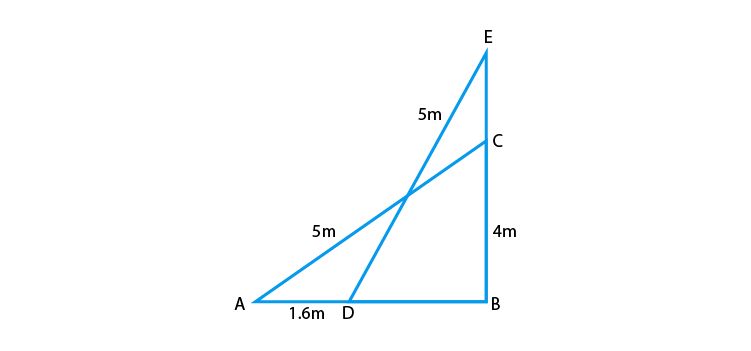# A 5 m long ladder is placed leaning towards a vertical wall such that it reaches the wall at a point 4 m high. If the foot of the ladder is moved 1.6 m towards the wall, then find the distance by which the top of the ladder would slide upwards on the wall.

Solution

Let the length of the ladder = AC = 5 m

Let the height of the wall on which ladder is placed = BC = 4m.From right-angled ∆EBD,

On applying the Pythagoras Theorem,

ED2 = EB2 + BD2

(5)2 = (EB)2 + (14)2 [ BD = 1.4]

25 = (EB)2 + 1.96

(EB)2 = 25 –1.96 = 23.04

EB = √23.04 = 4.8

Now, we have,

EC = EB – BC = 4.8 – 4 = 0.8

Hence, the top of the ladder would slide upwards on the wall by a distance of 0.8 m.(22)(5)